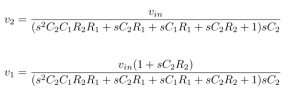# CIRCUIT ANALYSIS

CIRCUIT ANALYSIS:
Lets analysis the following circuit.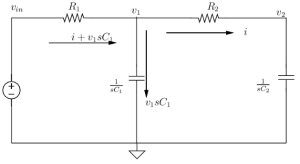This circuit consist of two nodes named as v1 & v2
Consider the input voltage is vin .
Lets say the current ‘i’ is passing through the node v2 .
Then the voltage at the node v2 is given by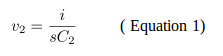Voltage at the node V1 is given by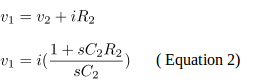Then the input voltage vin is given by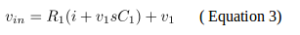Substituting the value of V1 in equation 3 we get,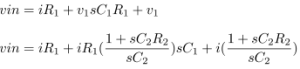(Equation 4)

Then the current ‘i’ passing through the node V1 is,(Equation 5)

The transfer function (by substituting the value of ‘i’ in equations 1 & 2)